Short and Long Answer Questions: Structure of the Atom

# Short and Long Answer Questions: Structure of the Atom - Science Class 9

### Very Short Answer Type Questions

Ques 1: Draw the atomic structure of the hydrogen atom.
Ans: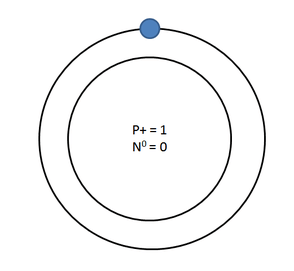Hydrogen Atom
K=1 electron (no neutron)
Ques 2: Why are some elements chemically inert?
Ans: Because their outermost shell is completely filled.

Ques 3: Why is an atom electrically neutral?
Ans: It has same number of protons and electrons, (positive charge = negative charge).

Ques 4: What is the charge and mass of a-particles?
Ans: Charge is + 2
Mass is 4 a.m.u.

Ques 5: What are valence electrons?
Ans: Electrons present in the outermost shell of an atom are called valence electrons.

Ques 6: An atom has atomic number 12, what is its valency and name the element?
Ans: Atomic number = 12
∴ Protons = Electrons = 12 Electrons Configuration = K L M -2 8 2
∴ Valency = 2
Element is magnesium.

Ques 7: Find the number of neutrons in 2713X.
Ans: Mass number = 27
∴ p + n = 27 p = 13, (Atomic No. = Number of protons)
∴ 13 + n = 27
∴ n = 14
∴ Neutron =14

Ques 8: Where is the mass of atom concentrated?
Ans: Mass of an atom is concentrated in nucleus.

Ques 9: Name two elements with same number of protons and neutrons?
Ans: Carbon (Protons = Neutrons = 6)
Oxygen (Protons = Neutrons = 8)

Ques 10: Draw the atomic structure of sodium atom.
Ans: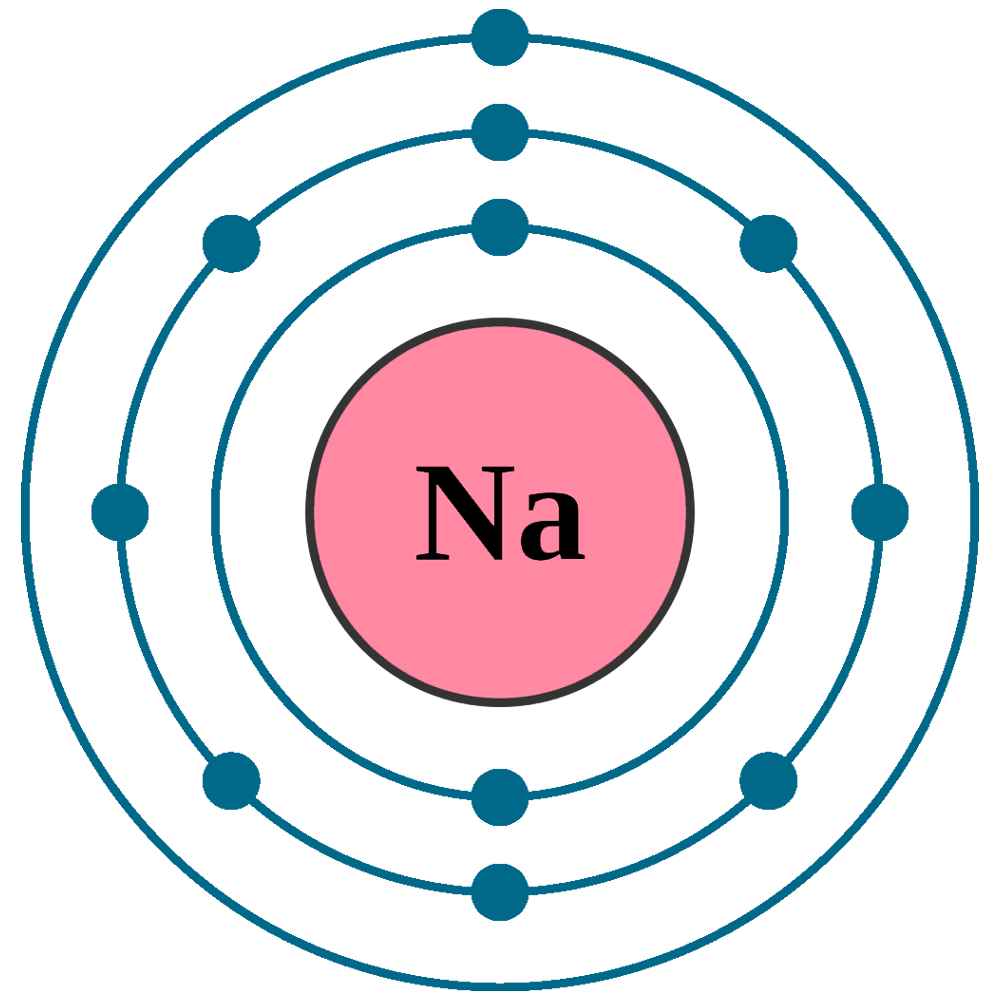Sodium Atom

Ques  11: Name the isotope used for the treatment of cancer.
Ans: Isotope of cobalt.

Ques 12: AZX What does this symbol represent?
Ans: X —> Symbol of element
A —> Mass number
Z —> Atomic number

Ques 13: Can the value of ‘Z’ be same for two different atoms?
Ans: No, (Z = atomic number), two different atoms cannot have same atomic number.

Ques 14: Can the value of A’ be same for two different atoms?
Ans: Yes, it can be e.g. Ca and Ar has A-40 (i.e., mass number).

Ques 1: Name the scientist who discovered protons and neutrons in an atoms.
Ans: Protons were discovered by E. Goldstein in 1866 and neutrons were discovered by J, Chadwick in 1932.

Ques 2: What is the contribution of Bohr and Bury together in the structure of atom’s explanation?
Ans: Both Bohr and Bury gave the distribution of electrons into different atoms by giving the formula 2n2, where n = shell number.

Ques 3: Draw the atomic structure of (i) an atom with same number of sub-atomic particles, (ii) an atom with same number of electrons in L and M shell.
Ans: (i) An atom with same number of sub-atomic particles is Helium
No. of protons = 2
No. of electrons = 2
No. of Neutrons = 2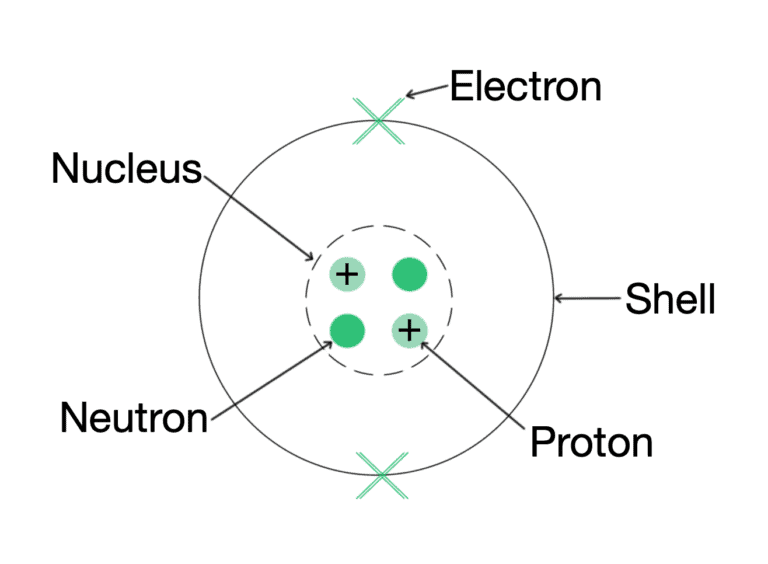The helium atom is having 2 electrons, 2 protons and 2 neutrons.

(ii) Argon is and atom with L and M shell filled —->K L M- 2, 8, 8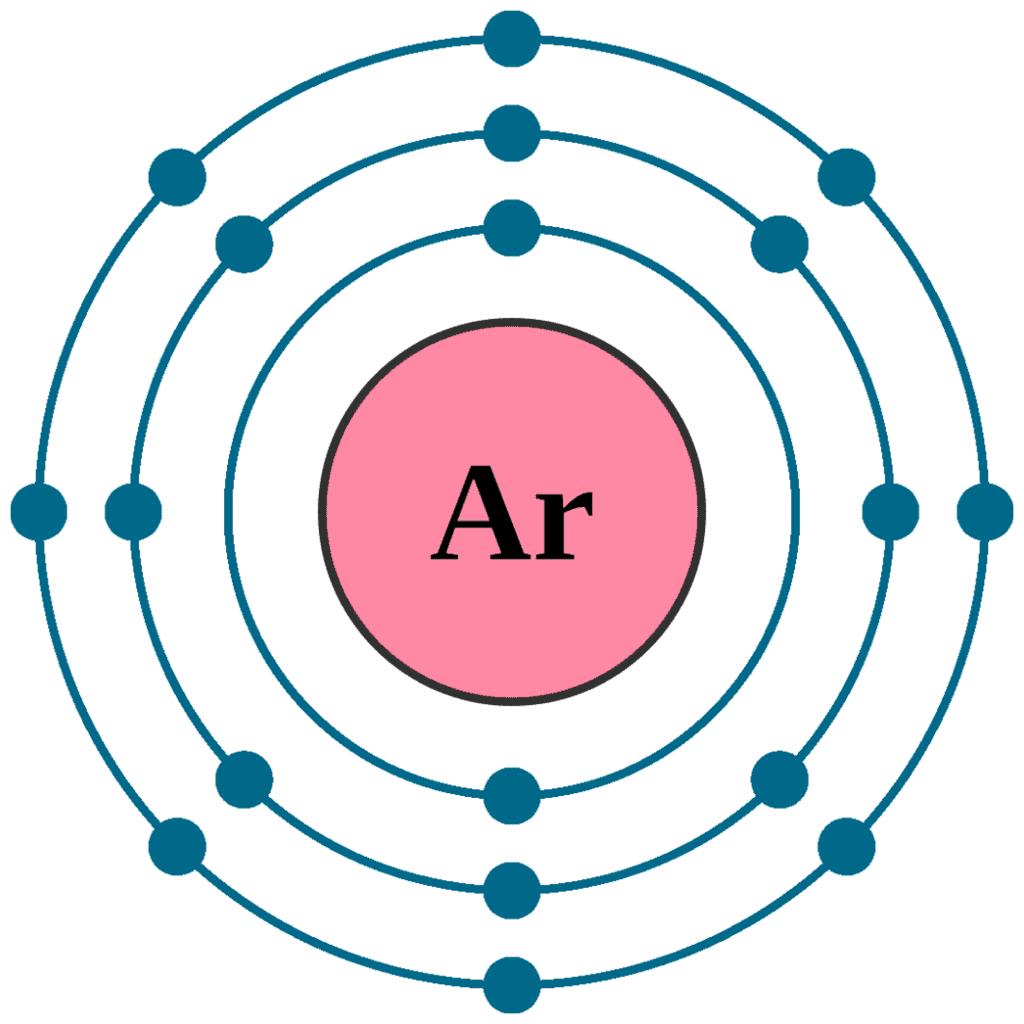Ques 4: What is an octate? Why would atoms want to complete their octate?
Ans: When the outermost shell of an atom i.e., L, M or N are completely filled with 8 electrons in the shell, it is said an octate. Atoms would want to complete their octate because they want to become stable.

Ques 5: Find the valency of 147N and 3517Cl.
Ans: The atomic number of nitrogen = 7, No. of protons = 7, No. of electrons = 7
Electronic configuration = K L M =2 5 –
Valency = 3
Because either it will gain three electrons or share 3 electrons to complete its octate.
The atomic number of chlorine = 17, p = 17, e=17
Electronic configuration = K L M= 2 8 7
Valency = 1
Because it will gain 1 electron to complete its octate.

Ques 6: Pick up the isotopes among the following and state reason.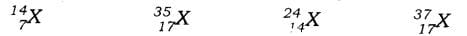Ans: The isotopes are 3517X and 3717X as both the atoms show the same atomic number but a different mass number.

Ques 7: Pick up atoms that have same number of neutrons from the following: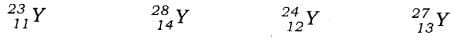Ans: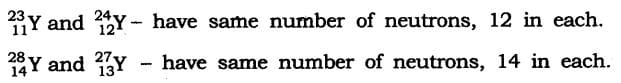Ques 8: What are nucleons? What is the name given to those atoms which have same number of nucleons in it?
Ans: Protons and neutrons present in the nucleus are called nucleons Isobaric elements have same number of nucleons in it.

 Element Protons Neutrons (Protons + Neutrons) Argon 18 22 40 Calcium 20 20 40 Potassium 19 21 40

Ques 9: Give the difference between three sub-atomic particles.
Ans: Three sub-atomic particles are electron, proton and neutron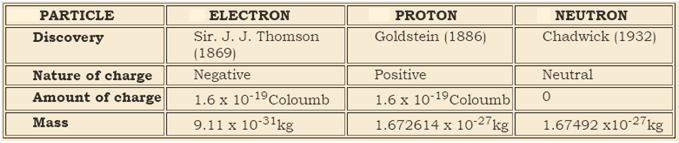Ques 10. Give the names of three atomic species of hydrogen.
Ans: Three atomic species of hydrogen are: Hydrogen, Deuterium, Tritum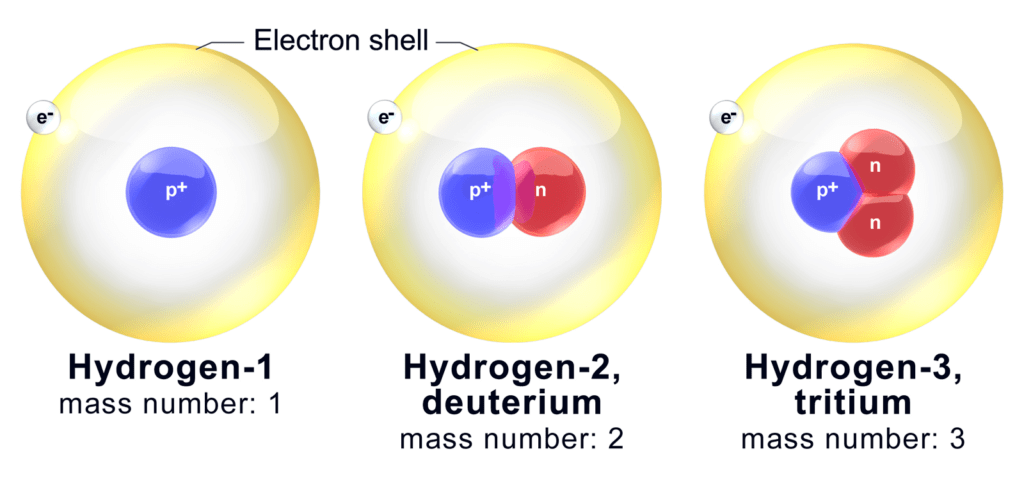Ques 11: Atomic Mass exists as a whole number, why do we write the atomic mass of chlorine as 35.5 u.
Ans: Chlorine has two isotopes and the mass of an atom is taken as the average mass of all the naturally occurring atoms of that element.
This is obtained by knowing the percentage of each isotopic from and then the average mass is calculated Cl = 35 - 75% and Cl = 37 - 25%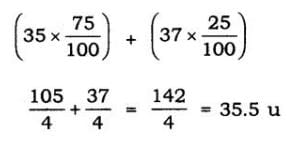Ques 12:  Give the difference between isotopes, and isobars.
Ans:

 Isotopes Isobars Are atoms of same element. Are atoms of different element Have same atomic number Have different atomic number Have different mass number Have same mass number Number of protons and electrons are same in these atoms. Number of protons and electrons are not  same in these atoms.

Ques 13: Number of protons and electrons are same in an atom. Then why is it wrong to say that atomic number of an atom is equal to its number of electrons.
Ans: Atomic number ≠ Number of electrons, although number of protons = number of electrons because the electron’s number can change in an atom by loss, or gain of it. But the proton’s number remains constant (as it does not take part in loss or gain).

Ques 14: An atom is electrically neutral, on loss or gain of electrons why does it become charged?
Ans: An atom is electrically neutral because of a same number of protons and electrons. But it becomes charged, to become a stable atom, loses or gains electrons. Hence,
Number of protons ≠ Number of electrons
If it loses electrons p > e; hence +ve charge is obtained.
If it gains electrons e > p; hence -ve charge is obtained.

Ques 15: What is valency? Explain different types of valencies.
Ans: The combining capacity of an atom is called its valency. There are 2 types of valencies.

 Covalency Electrovalency When an atom share electrons, they have combined capacity. When an atom loses or gains electrons

Some atoms also show zero valencies when their outermost shell is completely filled.

Ques 16:  With the help of activity in daily life, how can you prove that atoms are divisible.
Ans: Activity
• Take a scale, rub it on hair, try to attract a small bit of paper.
• Now divide the bit of paper further into smaller pieces.
• Again bring the charged scale near to these pieces of papers.
• You will observe that the bits of paper still get attracted.
Conclusion: This activity shows that the atom contains charges and these charges are opposite in nature which shows the attraction. Hence here scale and paper are oppositely charged and hence attract each other. Also, every atom has at least one sub-atomic particle.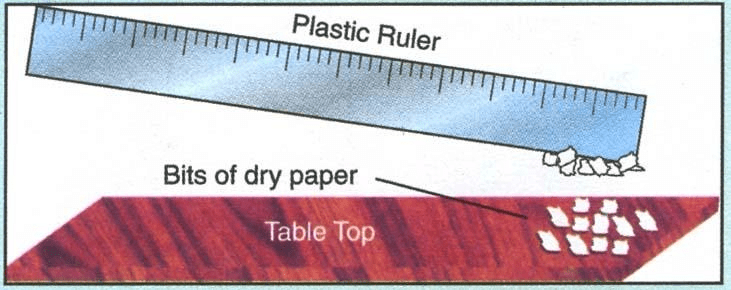Ques 17: In the structure of an atom why are protons present in the centre and are not pulled outside by the electrons as both are oppositely charged with the same unit of charge?
Ans: Protons are heavy with a mass 1 unit and hence are concentrated in the centre of the atom. The mass of electrons is negligible i.e.1/1800 times less than that of protons. Hence are not able to attract the protons and pull them out of the nucleus, although their charge is of the same value.

Ques 18: According to you, among the structure of atom studied which model is correct and why?
Ans: Bohr’s model of an atom is the best model and is correct because it gives the explanation of nucleons (protons and neutrons) in the centre and how electrons revolve around the nucleons in their discrete, special orbits, so electrons don’t loose/radiate energy and remain bonded in their shell.

Question 1. Give an activity to understand the implications of Rutherford’s alpha scattering experiment by a gold foil.
Ans: To understand the implications of Rutherford’s a-particle scattering experiment:
Activity: Let a child stand in front of a wall with his eyes closed. Let him throw stones at the wall from a distance. He will hear sound for each strike of stone on the wall. This is like the nucleus of the atom. But if a blindfolded child has to throw stones at a barbed-wire fence, most of the stones would not hit the fencing and no sound would be heard.
This is because there are lots of gap in the fence which allows the stone to pass through them. This is like empty space in an atom through which a-particles will pass through. Based on the above activity and similar reasoning Rutherford concluded the a-particle scattering experiment as:
(1) Most of the space inside the atom is empty as a-particles passed through the foil.
(2) Very few particles deflected from their path, this shows that positive charge occupies less space.
(3) A very small fraction of a-particles are deflected by 180°, this shows that all the positive charge and mass of the gold atom were concentrated in a very small volume within the atom.

Ques 2: What are isotopes? State its characteristics, give uses of isotopes?
Answer: Atoms of same element with same atomic number but different mass number are isotopes.
Characteristics:
(1) Physical properties of the isotopes are different e.g. mass, density.
(2) Chemical properties of the isotopes are same due to same number of electrons.
Uses:
(1) Uranium isotope is used as a fuel in nuclear reactor (U-235).
(2) Cobalt isotope is used for treatment of cancer (Co-60).
(3) Iodine isotope is used in the treatment of goitre.

Ques 3: Explain Rutherford’s α-particle scattering experiment and give its observation and conclusion drawn.
Ans: Rutherford’s α-particle scattering experiment:
Fast-moving α-particles were made to fall on a thin gold foil. Particles have + 2 charge and 4u mass, and considerable amount of energy.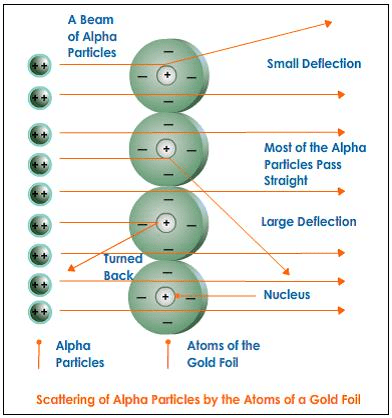Observations:
(1) Most of the α-particles passed straight through the foil.
(2) Some of the α-particles were deflected by small angles by the foil.
(3) One out of every 12000 particles rebounded.
The conclusion from observation:
(1) Most of the space inside the foil is empty.
(2) Positive charge of the atom occupies very little space.
(3) Mass of the atom is concentrated in the centre with all positive charge concentrated in a small volume within the atom.

Ques 4: Establish the relationship between atomic number, mass number, isotopes, isobars and valency of an atom.
Ans: Atomic number — Gives the number of protons (Z)
Mass number — Gives the number of protons and neutrons (A)
Isotopes — When atoms of the same element have the same number of protons (Z) but different number of neutrons (s) such atoms are called isotopes.
Isobars — When an atom of different elements have the same mass number (A) but different atomic number (Z) such atoms are called isobars.
Valency — It is the combining capacity of an atom.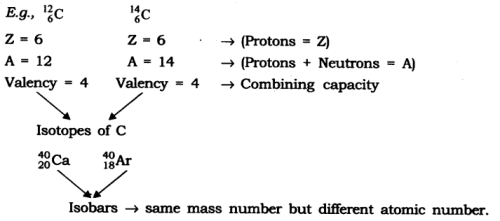The document Short and Long Answer Questions: Structure of the Atom | Science Class 9 is a part of the Class 9 Course Science Class 9.
All you need of Class 9 at this link: Class 9

## Science Class 9

66 videos|352 docs|97 tests

## FAQs on Short and Long Answer Questions: Structure of the Atom - Science Class 9

 1. What is the structure of an atom?Ans. The structure of an atom consists of a nucleus at the center, containing protons and neutrons, surrounded by electrons in energy levels or shells.
 2. What are the subatomic particles in an atom?Ans. The subatomic particles in an atom are protons, neutrons, and electrons. Protons have a positive charge, neutrons are neutral, and electrons have a negative charge.
 3. How are protons, neutrons, and electrons arranged in an atom?Ans. Protons and neutrons are located in the nucleus of an atom, while electrons are found in energy levels or shells surrounding the nucleus.
 4. What is the role of protons, neutrons, and electrons in an atom?Ans. Protons and neutrons contribute to the mass of the atom and are responsible for its stability. Electrons determine the chemical properties and reactivity of the atom.
 5. How does the structure of an atom relate to its properties?Ans. The number of protons determines the atomic number and identity of the element. The arrangement and number of electrons dictate the atom's chemical behavior and bonding capabilities. The presence of neutrons affects the atom's stability and isotopic variations. Overall, the structure of an atom directly influences its physical and chemical properties.

## Science Class 9

66 videos|352 docs|97 testsExplore Courses for Class 9 examSignup to see your scores go up within 7 days! Learn & Practice with 1000+ FREE Notes, Videos & Tests.
10M+ students study on EduRev
Track your progress, build streaks, highlight & save important lessons and more!
Related Searches

,

,

,

,

,

,

,

,

,

,

,

,

,

,

,

,

,

,

,

,

,

;